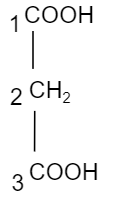# IUPAC name of the formula $C{{H}_{2}}{{(COOH)}_{2}}$ is?A. Butanoic acidB. $\text{Propane}-1,3-\text{dioic acid}$ C. $\text{Propane}-1,2-\text{dioic acid}$ D. Malonic acidVerified
177.6k+ views
Hint: We should know that IUPAC name is determined by the number of carbon atoms present in the hydrocarbon chain. So, first count the atoms of carbon in the given formula and give the name according to the number of carbons. Then define the IUPAC name by what type of group is attached in the branch of the chain.

So, here we can see it has three carbon atoms in the given formula $C{{H}_{2}}{{(COOH)}_{2}}$. So, it will be known by the name propane (as it has single covalent bonds attached to the side groups with the carbon atoms). And we can see there are two carboxylic acids. So, the suffix will be known by dioic. The carboxylic acids are present in the first and third carbon atom. So, the IUPAC name will be $\text{Propane}-1,3-\text{dioic acid}$ and its structure is shown as below,The possible mistake could be you can get confused with option D i.e. malonic acid. The given formula with IUPAC name $\text{Propane}-1,3-\text{dioic acid}$ is also known by the common name “malonic acid”. Remember, the common name and IUPAC nomenclature are two different entities. IUPAC nomenclature is a method which depends on the chain of carbon atoms and the group attached to it.